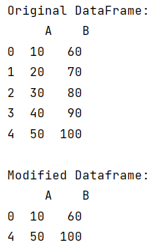# Extract first and last row of a DataFrame in Pandas

Given a Pandas DataFrame, we have to extract first and last row. By Pranit Sharma Last updated : September 23, 2023

Pandas is a special tool that allows us to perform complex manipulations of data effectively and efficiently. Inside pandas, we mostly deal with a dataset in the form of DataFrame. DataFrames are 2-dimensional data structures in pandas. DataFrames consist of rows, columns, and data.

## Problem statement

Given a Pandas DataFrame, we have to extract first and last row.

## Extracting first and last row of a DataFrame in Pandas

For this purpose, we will use the pandas.DataFrame.iloc property. 'i' in iloc stands for 'index'. This is also a data selection method but here, we need to pass the proper index as a parameter to select the required row or column. Index is nothing but the integer value ranging from 0 to n-1 which represents the number of rows or columns. We can perform various operations using the pandas.DataFrame.iloc property. Inside pandas.DataFrame.iloc property, the index value of the row comes first followed by the number of columns.

Note

To work with pandas, we need to import pandas package first, below is the syntax:

```import pandas as pd
```

Let us understand with the help of an example,

## Python program to extract first and last row of a DataFrame in Pandas

```# Importing pandas package
import pandas as pd

# Creating a dictionary
d = {
'A':[10,20,30,40,50],
'B':[60,70,80,90,100]
}

# Creating a DataFrame
df = pd.DataFrame(d)

# Display original DataFrame
print("Original DataFrame:\n",df,"\n")

# Extracting first and last row of dataframe
result = df.iloc[[0, -1]]

# # Display result
print("Result:\n",result)
```

### Output

The output of the above program is: